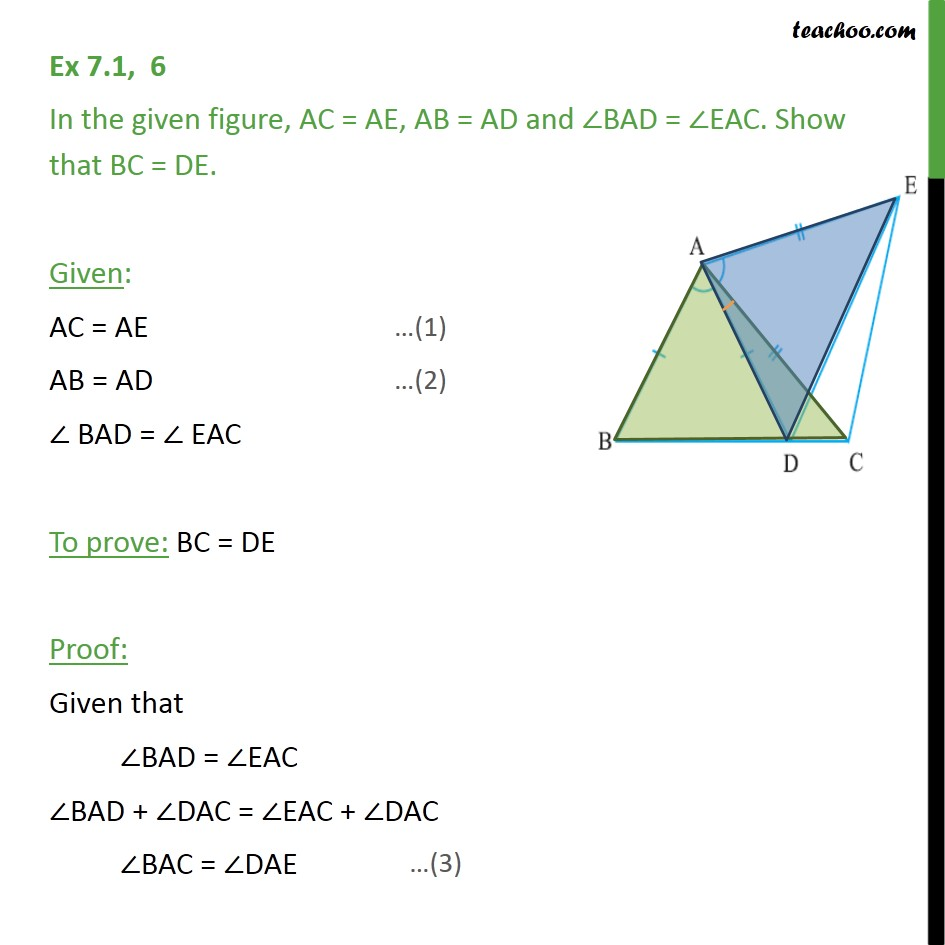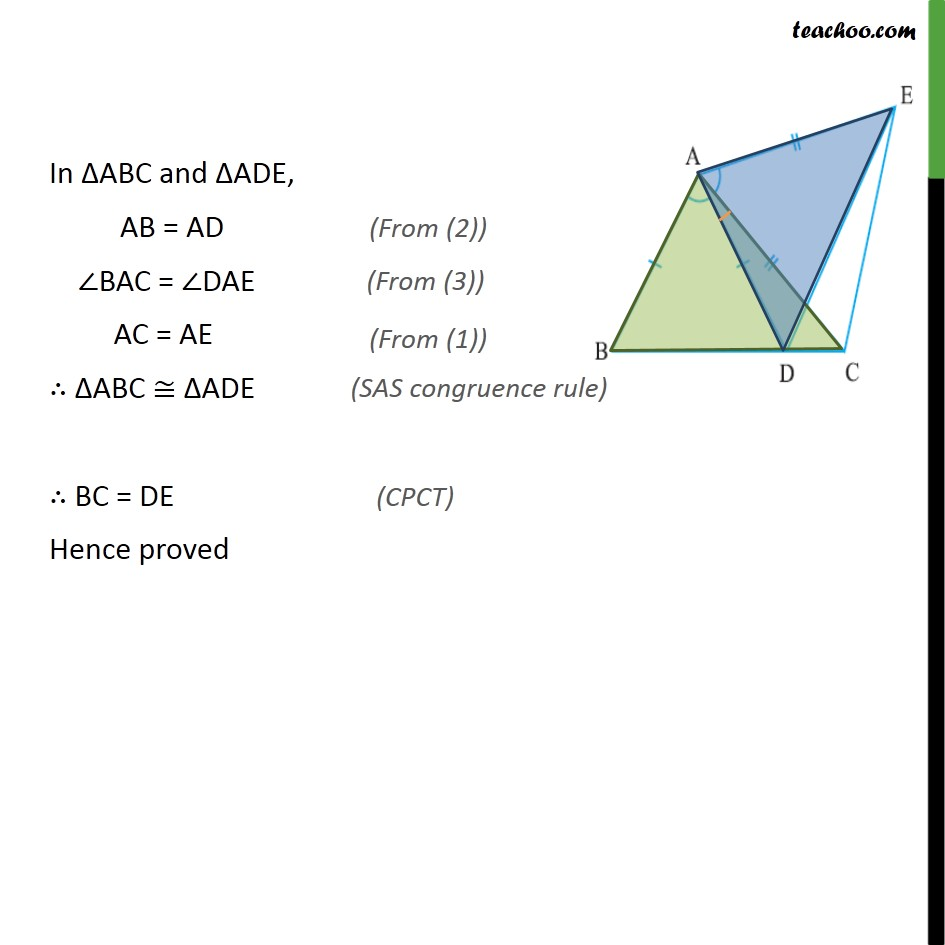Ex 7.1

Chapter 7 Class 9 Triangles
Serial order wiseLearn in your speed, with individual attention - Teachoo Maths 1-on-1 Class

### Transcript

Ex 7.1, 6 In the given figure, AC = AE, AB = AD and BAD = EAC. Show that BC = DE. Given: AC = AE AB = AD BAD = EAC To prove: BC = DE Proof: Given that BAD = EAC BAD + DAC = EAC + DAC BAC = DAE In ABC and ADE, AB = AD BAC = DAE AC = AE ABC ADE BC = DE Hence proved